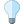#### Versions

You are viewing the RapidMiner Studio documentation for version 8.2 - Check here for latest version

#Linear Regression (RapidMiner Studio Core)

## Synopsis

This operator calculates a linear regression model from the input ExampleSet.

## Description

Regression is a technique used for numerical prediction. Regression is a statistical measure that attempts to determine the strength of the relationship between one dependent variable ( i.e. the label attribute) and a series of other changing variables known as independent variables (regular attributes). Just like Classification is used for predicting categorical labels, Regression is used for predicting a continuous value. For example, we may wish to predict the salary of university graduates with 5 years of work experience, or the potential sales of a new product given its price. Regression is often used to determine how much specific factors such as the price of a commodity, interest rates, particular industries or sectors influence the price movement of an asset.

Linear regression attempts to model the relationship between a scalar variable and one or more explanatory variables by fitting a linear equation to observed data. For example, one might want to relate the weights of individuals to their heights using a linear regression model.

This operator calculates a linear regression model. It uses the Akaike criterion for model selection. The Akaike information criterion is a measure of the relative goodness of a fit of a statistical model. It is grounded in the concept of information entropy, in effect offering a relative measure of the information lost when a given model is used to describe reality. It can be said to describe the tradeoff between bias and variance in model construction, or loosely speaking between accuracy and complexity of the model.

## Differentiation

###Polynomial Regression

Polynomial regression is a form of linear regression in which the relationship between the independent variable x and the dependent variable y is modeled as an nth order polynomial.

## Input

•training set (Data Table)

This input port expects an ExampleSet. This operator cannot handle nominal attributes; it can be applied on data sets with numeric attributes. Thus often you may have to use the Nominal to Numerical operator before application of this operator.

## Output

•model (Linear Regression Model)

The regression model is delivered from this output port. This model can now be applied on unseen data sets.

•example set (Data Table)

The ExampleSet that was given as input is passed without changing to the output through this port. This is usually used to reuse the same ExampleSet in further operators or to view the ExampleSet in the Results Workspace.

•weights (Attribute Weights)

This port delivers the attribute weights.

## Parameters

• feature_selectionThis is an expert parameter. It indicates the feature selection method to be used during regression. Following options are available: none, M5 prime, greedy, T-Test, iterative T-Test Range: selection
• alphaThis parameter is available only when the feature selection parameter is set to 'T-Test'. It specifies the value of alpha to be used in the T-Test feature selection. Range: real
• max_iterationsThis parameter is only available when the feature selection parameter is set to 'iterative T-Test'. It specifies the maximum number of iterations of the iterative T-Test for feature selection. Range: integer
• forward_alphaThis parameter is only available when the feature selection parameter is set to 'iterative T-Test'. It specifies the value of forward alpha to be used in the T-Test feature selection. Range: real
• backward_alphaThis parameter is only available when the feature selection parameter is set to 'iterative T-Test'. It specifies the value of backward alpha to be used in the T-Test feature selection. Range: real
• eliminate_colinear_featuresThis parameter indicates if the algorithm should try to delete collinear features during the regression or not. Range: boolean
• min_toleranceThis parameter is only available when the eliminate colinear features parameter is set to true. It specifies the minimum tolerance for eliminating collinear features. Range: real
• use_biasThis parameter indicates if an intercept value should be calculated or not. Range: boolean
• ridgeThis parameter specifies the ridge parameter for using in ridge regression. Range: real

## Tutorial Processes

### Applying the Linear Regression operator on the Polynomial data set

The 'Polynomial' data set is loaded using the Retrieve operator. The Filter Example Range operator is applied on it. The first example parameter of the Filter Example Range parameter is set to 1 and the last example parameter is set to 100. Thus the first 100 examples of the 'Polynomial' data set are selected. The Linear Regression operator is applied on it with default values of all parameters. The regression model generated by the Linear Regression operator is applied on the last 100 examples of the 'Polynomial' data set using the Apply Model operator. Labeled data from the Apply Model operator is provided to the Performance (Regression) operator. The absolute error and the prediction average parameters are set to true. Thus the Performance Vector generated by the Performance (Regression) operator has information regarding the absolute error and the prediction average in the labeled data set. The absolute error is calculated by adding the difference of all predicted values from the actual values of the label attribute, and dividing this sum by the total number of predictions. The prediction average is calculated by adding all actual label values and dividing this sum by the total number of examples. You can verify this from the results in the Results Workspace.